# mesh¶

Submodule to manage polygonal meshes.

## Mesh¶

class vedo.mesh.Mesh(inputobj=None, c=None, alpha=1, computeNormals=False)[source]

Bases: vtkRenderingCorePython.vtkFollower, vedo.base.BaseActor

Build an instance of object Mesh derived from vtkActor.

Input can be vtkPolyData, vtkActor, or a python list of [vertices, faces].

If input is any of vtkUnstructuredGrid, vtkStructuredGrid or vtkRectilinearGrid the geometry is extracted. In this case the original VTK data structures can be accessed with: mesh.inputdata().

Finally input can be a list of vertices and their connectivity (faces of the polygonal mesh). For point clouds - e.i. no faces - just substitute the faces list with None.

E.g.: Mesh( [ [[x1,y1,z1],[x2,y2,z2], …], [[0,1,2], [1,2,3], …] ] )

Parameters
• c – color in RGB format, hex, symbol or name

• alpha (float) – opacity value

• wire (bool) – show surface as wireframe

• bc – backface color of internal surface

• texture (str) – jpg file name or surface texture name

• computeNormals (bool) – compute point and cell normals at creation

Hint

A mesh can be built from vertices and their connectivity. See e.g.:

addCurvatureScalars(method=0, lut=None)[source]

Add scalars to Mesh that contains the curvature calculated in three different ways.

Parameters
• method (int) – 0-gaussian, 1-mean, 2-max, 3-min curvature.

• lut – optional vtkLookUpTable up table.

Example
from vedo import Torus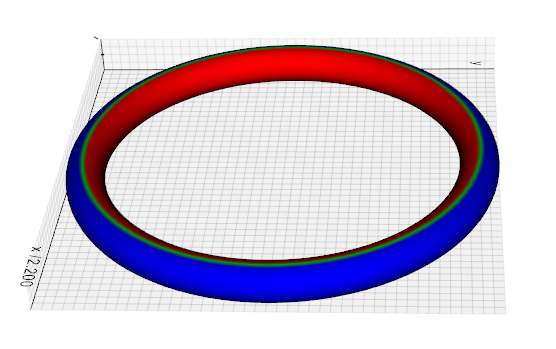addElevationScalars(lowPoint=(), highPoint=(), vrange=(), lut=None)[source]

Add to Mesh a scalar array that contains distance along a specified direction.

Parameters
• low (list) – one end of the line (small scalar values). Default (0,0,0).

• high (list) – other end of the line (large scalar values). Default (0,0,1).

• vrange (list) – set the range of the scalar. Default is (0, 1).

• lut – optional vtkLookUpTable up table (see makeLUT method).

Example
from vedo import Sphere

|elevation|

addShadow(x=None, y=None, z=None, c=0.5, 0.5, 0.5, alpha=1)[source]

Generate a shadow out of an Mesh on one of the three Cartesian planes. The output is a new Mesh representing the shadow. This new mesh is accessible through mesh.shadow. By default the shadow mesh is placed on the bottom/back wall of the bounding box.

Parameters

x,y,z (float) – identify the plane to cast the shadow to [‘x’, ‘y’ or ‘z’]. The shadow will lay on the orthogonal plane to the specified axis at the specified value of either x, y or z.

addTrail(offset=None, maxlength=None, n=50, c=None, alpha=None, lw=2)[source]

Add a trailing line to mesh. This new mesh is accessible through mesh.trail.

Parameters
• offset – set an offset vector from the object center.

• maxlength – length of trailing line in absolute units

• n – number of segments to control precision

• lw – line width of the trail

alpha(opacity=None)[source]

Set/get mesh’s transparency. Same as mesh.opacity().

applyTransform(transformation)[source]

Apply a linear or non-linear transformation to the mesh polygonal data.

Parameters

transformation – thevtkTransform or vtkMatrix4x4 objects.

area(value=None)[source]

Get/set the surface area of mesh.

averageSize()[source]

Calculate the average size of a mesh. This is the mean of the vertex distances from the center of mass.

backColor(bc=None)[source]

Set/get mesh’s backface color.

backFaceCulling(value=True)[source]

Set culling of polygons based on orientation of normal with respect to camera.

bc(backColor=False)[source]

Shortcut for mesh.backColor().

boundaries(boundaryEdges=True, featureAngle=65, nonManifoldEdges=True, returnPointIds=False, returnCellIds=False)[source]

Return a Mesh that shows the boundary lines of an input mesh.

Parameters
• boundaryEdges (bool) – Turn on/off the extraction of boundary edges.

• featureAngle (float) – Specify the feature angle for extracting feature edges.

• nonManifoldEdges (bool) – Turn on/off the extraction of non-manifold edges.

• returnPointIds (bool) – return a numpy array of point indices

• returnCellIds (bool) – return a numpy array of cell indices

cap(returnCap=False)[source]

Generate a “cap” on a clipped mesh, or caps sharp edges.

cellColors(input_array=None, cmap='jet', alpha=1, vmin=None, vmax=None, arrayName='CellScalars')[source]

Set individual cell colors by providing a list of scalar values and a color map. scalars can be a string name of the vtkArray.

Parameters
• alphas (list) – single value or list of transparencies for each vertex

• cmap (str, list, vtkLookupTable, matplotlib.colors.LinearSegmentedColormap) – color map scheme to transform a real number into a color.

• alpha (float, list) – mesh transparency. Can be a list of values one for each vertex.

• vmin (float) – clip scalars to this minimum value

• vmax (float) – clip scalars to this maximum value

• arrayName (str) – give a name to the array

cellIndividualColors(colorlist, alpha=1, alphaPerCell=False)[source]

Colorize the faces of a mesh one by one, passing a 1-to-1 list of colors and optionally a list of transparencies.

Parameters

alphaPerCell (bool) –

Only matters if alpha is a sequence. If so: if True assume that the list of opacities is independent on the colors (same color cells can have different opacity), this can be very slow for large meshes,

if False [default] assume that the alpha matches the color list (same color has the same opacity). This is very fast even for large meshes.

centerOfMass()[source]

Get the center of mass of mesh.

clean(tol=None)[source]

Clean mesh polydata. Can also be used to decimate a mesh if tol is large. If tol=None only removes coincident points.

Parameters

tol – defines how far should be the points from each other in terms of fraction of the bounding box length.

clone()[source]

Clone a Mesh object to make an exact copy of it.

closestPoint(pt, N=1, radius=None, returnIds=False)[source]

Find the closest point(s) on a mesh given from the input point pt.

Parameters
• N (int) – if greater than 1, return a list of N ordered closest points.

• radius (float) – if given, get all points within that radius.

• returnIds (bool) – return points IDs instead of point coordinates.

Note

The appropriate kd-tree search locator is built on the fly and cached for speed.

color(c=False)[source]

Set/get mesh’s color. If None is passed as input, will use colors from active scalars. Same as mesh.c().

computeNormals(points=True, cells=True)[source]

Compute cell and vertex normals for the mesh.

Warning

Mesh gets modified, output can have a different nr. of vertices.

computeNormalsWithPCA(n=20, orientationPoint=None, flip=False)[source]

Generate point normals using PCA (principal component analysis). Basically this estimates a local tangent plane around each sample point p by considering a small neighborhood of points around p, and fitting a plane to the neighborhood (via PCA).

Parameters
• n (int) – neighborhood size to calculate the normal

• orientationPoint (list) – adjust the +/- sign of the normals so that the normals all point towards a specified point. If None, perform a traversal of the point cloud and flip neighboring normals so that they are mutually consistent.

• flip (bool) – flip all normals

connectedCells(index, returnIds=False)[source]

Find all cellls connected to an input vertex specified by its index.

connectedVertices(index, returnIds=False)[source]

Find all vertices connected to an input vertex specified by its index.

Parameters

returnIds (bool) – return vertex IDs instead of vertex coordinates.

crop(top=None, bottom=None, right=None, left=None, front=None, back=None, bounds=None)[source]

Crop an Mesh object.

Parameters
• top (float) – fraction to crop from the top plane (positive z)

• bottom (float) – fraction to crop from the bottom plane (negative z)

• front (float) – fraction to crop from the front plane (positive y)

• back (float) – fraction to crop from the back plane (negative y)

• right (float) – fraction to crop from the right plane (positive x)

• left (float) – fraction to crop from the left plane (negative x)

• bounds (list) – direct list of bounds passed as [x0,x1, y0,y1, z0,z1]

Example

from vedo import Sphere
Sphere().crop(right=0.3, left=0.1).show()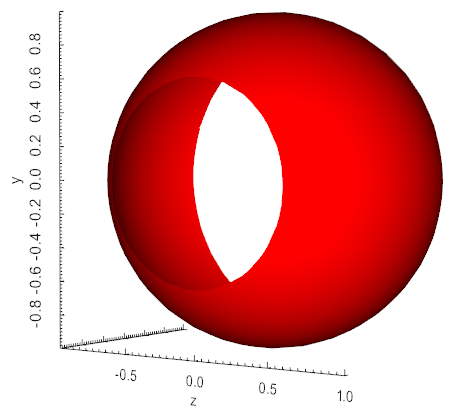cutWithMesh(mesh, invert=False)[source]

Cut an Mesh mesh with another Mesh.

Parameters

invert (bool) – if True return cut off part of Mesh.

cutWithPlane(origin=0, 0, 0, normal=1, 0, 0, returnCut=False)[source]

Cut the mesh with the plane defined by a point and a normal.

Parameters
• origin – the cutting plane goes through this point

• normal – normal of the cutting plane

Example
from vedo import Cube

cube = Cube().cutWithPlane(normal=(1,1,1))
cube.bc('pink').show()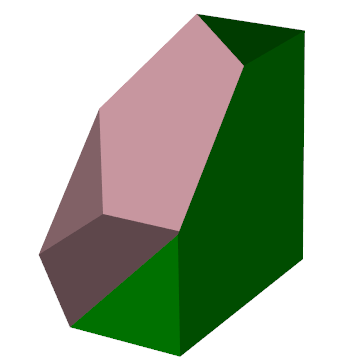cutWithPointLoop(points, invert=False)[source]

Cut an Mesh object with a set of points forming a closed loop.

decimate(fraction=0.5, N=None, method='quadric', boundaries=False)[source]

Downsample the number of vertices in a mesh to fraction.

Parameters
• fraction (float) – the desired target of reduction.

• N (int) – the desired number of final points (fraction is recalculated based on it).

• method (str) – can be either ‘quadric’ or ‘pro’. In the first case triagulation will look like more regular, irrespective of the mesh origianl curvature. In the second case triangles are more irregular but mesh is more precise on more curved regions.

• boundaries (bool) – (True), in pro mode decide whether to leave boundaries untouched or not.

Note

Setting fraction=0.1 leaves 10% of the original nr of vertices.

deletePoints(indices, renamePoints=False)[source]

Delete a list of vertices identified by their index.

Parameters

renamePoints (bool) – if True, point indices and faces are renamed. If False, vertices are not really deleted and faces indices will stay unchanged (default, faster).

distanceToMesh(mesh, signed=False, negate=False)[source]

Computes the (signed) distance from one mesh to another.

extractLargestRegion()[source]

Extract the largest connected part of a mesh and discard all the smaller pieces.

extrude(zshift=1, rotation=0, dR=0, cap=True, res=1)[source]

Sweep a polygonal data creating a “skirt” from free edges and lines, and lines from vertices. The input dataset is swept around the z-axis to create new polygonal primitives. For example, sweeping a line results in a cylindrical shell, and sweeping a circle creates a torus.

You can control whether the sweep of a 2D object (i.e., polygon or triangle strip) is capped with the generating geometry. Also, you can control the angle of rotation, and whether translation along the z-axis is performed along with the rotation. (Translation is useful for creating “springs”). You also can adjust the radius of the generating geometry using the “dR” keyword.

The skirt is generated by locating certain topological features. Free edges (edges of polygons or triangle strips only used by one polygon or triangle strips) generate surfaces. This is true also of lines or polylines. Vertices generate lines.

This filter can be used to model axisymmetric objects like cylinders, bottles, and wine glasses; or translational/rotational symmetric objects like springs or corkscrews.

Warning

Some polygonal objects have no free edges (e.g., sphere). When swept, this will result in two separate surfaces if capping is on, or no surface if capping is off.

faces()[source]

Get cell polygonal connectivity ids as a python list. The output format is: [[id0 … idn], [id0 … idm], etc].

fillHoles(size=None)[source]

Identifies and fills holes in input mesh. Holes are identified by locating boundary edges, linking them together into loops, and then triangulating the resulting loops.

Parameters

size (float) – approximate limit to the size of the hole that can be filled.

Example: fillholes.py

flat()[source]

Set surface interpolation to Flat.flipNormals()[source]

Flip all mesh normals. Same as mesh.mirror(‘n’).

followCamera(cam=None)[source]

Mesh object will follow camera movements and stay locked to it.

Parameters

cam (vtkCamera) – if None the text will auto-orient itself to the active camera. A vtkCamera object can also be passed.

frontFaceCulling(value=True)[source]

Set culling of polygons based on orientation of normal with respect to camera.

insidePoints(pts, invert=False, tol=1e-05, returnIds=False)[source]

Return the point cloud that is inside mesh surface.

intersectWithLine(p0, p1)[source]

Return the list of points intersecting the mesh along the segment defined by two points p0 and p1.

Example
from vedo import *
s = Spring(alpha=0.2)
pts = s.intersectWithLine([0,0,0], [1,0.1,0])
ln = Line([0,0,0], [1,0.1,0], c='blue')
ps = Points(pts, r=10, c='r')
show(s, ln, ps, bg='white')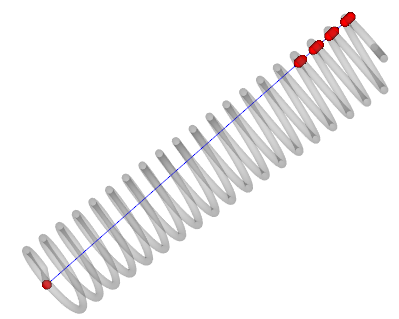isInside(point, tol=0.0001)[source]

Return True if point is inside a polydata closed surface.

isobands(n=10, vmin=None, vmax=None)[source]

Return a new Mesh representing the isobands of the active scalars. This is a new mesh where the scalar is now associated to cell faces and used to colorize the mesh.

Parameters
• n (int) – number of isolines in the range

• vmin (float) – minimum of the range

• vmax (float) – maximum of the range

isolines(n=10, vmin=None, vmax=None)[source]

Return a new Mesh representing the isolines of the active scalars.

Parameters
• n (int) – number of isolines in the range

• vmin (float) – minimum of the range

• vmax (float) – maximum of the range

join(polys=True)[source]

Generate triangle strips and/or polylines from input polygons, triangle strips, and lines.

Input polygons are assembled into triangle strips only if they are triangles; other types of polygons are passed through to the output and not stripped. Use mesh.triangulate() to triangulate non-triangular polygons prior to running this filter if you need to strip all the data.

Also note that if triangle strips or polylines are present in the input they are passed through and not joined nor extended. If you wish to strip these use mesh.triangulate() to fragment the input into triangles and lines prior to applying strip().

Parameters

polys (bool) – polygonal segments will be joined if they are contiguous

Warning

If triangle strips or polylines exist in the input data they will be passed through to the output data. This filter will only construct triangle strips if triangle polygons are available; and will only construct polylines if lines are available.

labels(content=None, cells=False, scale=None, rotX=0, rotY=0, rotZ=0, ratio=1, precision=3)[source]

Generate value or ID labels for mesh cells or points.

Parameters
• content (list,int,str) – either ‘id’, array name or array number. A array can also be passed (must match the nr. of points or cells).

• cells (bool) – generate labels for cells instead of points [False]

• scale (float) – absolute size of labels, if left as None it is automatic

• rotX (float) – local rotation angle of label in degrees

• ratio (int) – skipping ratio, to reduce nr of labels for large meshes

• precision (int) – numeric precision of labels

Example
from vedo import *
s = Sphere(alpha=0.2, res=10).lineWidth(0.1)
s.computeNormals().clean()
point_ids = s.labels(cells=False).c('green')
cell_ids  = s.labels(cells=True ).c('black')
show(s, point_ids, cell_ids)

lc(lineColor=None)[source]

Set/get color of mesh edges. Same as lineColor().

lineColor(lc=None)[source]

Set/get color of mesh edges. Same as lc().

lineWidth(lw=None)[source]

Set/get width of mesh edges. Same as lw().

lines(flat=False)[source]

Get lines connectivity ids as a numpy array. Default format is [[id0,id1], [id3,id4], …]

Parameters

flat (bool) – 1D numpy array as [2, 10,20, 3, 10,11,12, 2, 70,80, …]

lw(lineWidth=None)[source]

Set/get width of mesh edges. Same as lineWidth().

mirror(axis='x')[source]

Mirror the mesh along one of the cartesian axes.

normalAt(i)[source]

Return the normal vector at vertex point i.

normalize()[source]

Shift mesh center of mass at origin and scale its average size to unit.

normals(cells=False, compute=True)[source]

Retrieve vertex normals as a numpy array.

Params bool cells

if True return cell normals.

Params bool compute

if True normals are recalculated if not already present. Note that this might modify the number of mesh points.

opacity(alpha=None)[source]

Set/get mesh’s transparency. Same as mesh.alpha().

phong()[source]

Set surface interpolation to Phong.

pointColors(input_array=None, cmap='jet', alpha=1, vmin=None, vmax=None, arrayName='PointScalars')[source]

Set individual point colors by providing a list of scalar values and a color map. scalars can be a string name of the vtkArray.

Parameters
• alphas (list) – single value or list of transparencies for each vertex

• cmap (str, list, vtkLookupTable, matplotlib.colors.LinearSegmentedColormap) – color map scheme to transform a real number into a color.

• alpha (float, list) – mesh transparency. Can be a list of values one for each vertex.

• vmin (float) – clip scalars to this minimum value

• vmax (float) – clip scalars to this maximum value

• arrayName (str) – give a name to the array

pointGaussNoise(sigma)[source]

Add gaussian noise to point positions.

Parameters

sigma (float) – sigma is expressed in percent of the diagonal size of mesh.

Example
from vedo import Sphere


pointSize(ps=None)[source]

Set/get mesh’s point size of vertices. Same as mesh.ps()

points(pts=None, transformed=True, copy=False)[source]

Set/Get the vertex coordinates of the mesh. Argument can be an index, a set of indices or a complete new set of points to update the mesh.

Parameters
• transformed (bool) – if False ignore any previous transformation applied to the mesh.

• copy (bool) – if False return the reference to the points so that they can be modified in place, otherwise a copy is built.

polydata(transformed=True)[source]

Returns the vtkPolyData object of a Mesh.

Note

If transformed=True returns a copy of polydata that corresponds to the current mesh’s position in space.

projectOnPlane(direction='z')[source]

Project the mesh on one of the Cartesian planes.

ps(pointSize=None)[source]

Set/get mesh’s point size of vertices. Same as mesh.pointSize()

quality(measure=6, cmap='RdYlBu')[source]

Calculate functions of quality of the elements of a triangular mesh. See class vtkMeshQuality for explanation.

Parameters

measure (int) –

type of estimator

• EDGE RATIO, 0

• ASPECT RATIO, 1

• ASPECT FROBENIUS, 3

• MED ASPECT_FROBENIUS, 4

• MAX ASPECT FROBENIUS, 5

• MIN_ANGLE, 6

• COLLAPSE RATIO, 7

• MAX ANGLE, 8

• CONDITION, 9

• SCALED JACOBIAN, 10

• SHEAR, 11

• RELATIVE SIZE SQUARED, 12

• SHAPE, 13

• SHAPE AND SIZE, 14

• DISTORTION, 15

• MAX EDGE RATIO, 16

• SKEW, 17

• TAPER, 18

• VOLUME, 19

• STRETCH, 20

• DIAGONAL, 21

• DIMENSION, 22

• ODDY, 23

• SHEAR AND SIZE, 24

• JACOBIAN, 25

• WARPAGE, 26

• ASPECT GAMMA, 27

• AREA, 28

• ASPECT BETA, 29

quantize(binSize)[source]

The user should input binSize and all {x,y,z} coordinates will be quantized to that absolute grain size.

Example

from vedo import Paraboloid
Paraboloid().lw(0.1).quantize(0.1).show()

reverse(cells=True, normals=False)[source]

Reverse the order of polygonal cells and/or reverse the direction of point and cell normals. Two flags are used to control these operations:

• cells=True reverses the order of the indices in the cell connectivity list.

• normals=True reverses the normals by multiplying the normal vector by -1

(both point and cell normals, if present).

shear(x=0, y=0, z=0)[source]

Apply a shear deformation to the Mesh along one of the main axes. The transformation resets position and rotations so it should be applied first.

shrink(fraction=0.85)[source]

Shrink the triangle polydata in the representation of the input mesh.

Example

from vedo import *
s = Sphere(r=0.2).pos(0,0,-0.5)
show(pot, s)

silhouette(direction=None, borderEdges=True, featureAngle=False)[source]

Return a new line Mesh which corresponds to the outer silhouette of the input as seen along a specified direction, this can also be a vtkCamera object.

Parameters
• direction (list) – viewpoint direction vector. If None this is guessed by looking at the minimum of the sides of the bounding box.

• borderEdges (bool) – enable or disable generation of border edges

• featureAngle (float) – minimal angle for sharp edges detection. If set to False the functionality is disabled.

smoothLaplacian(niter=15, relaxfact=0.1, edgeAngle=15, featureAngle=60)[source]

Adjust mesh point positions using Laplacian smoothing.

Parameters
• niter (int) – number of iterations.

• relaxfact (float) – relaxation factor. Small relaxfact and large niter are more stable.

• edgeAngle (float) – edge angle to control smoothing along edges (either interior or boundary).

• featureAngle (float) – specifies the feature angle for sharp edge identification.

smoothMLS1D(f=0.2, radius=None)[source]

Smooth mesh or points with a Moving Least Squares variant. The list mesh.info['variances'] contain the residue calculated for each point. Input mesh’s polydata is modified.

Parameters
• f (float) – smoothing factor - typical range is [0,2].

• radius (float) – radius search in absolute units. If set then f is ignored.

smoothMLS2D(f=0.2, radius=None)[source]

Smooth mesh or points with a Moving Least Squares algorithm variant. The list mesh.info['variances'] contains the residue calculated for each point.

Parameters
• f (float) – smoothing factor - typical range is [0,2].

• radius (float) – radius search in absolute units. If set then f is ignored.

smoothWSinc(niter=15, passBand=0.1, edgeAngle=15, featureAngle=60)[source]

Adjust mesh point positions using the Windowed Sinc function interpolation kernel.

Parameters
• niter (int) – number of iterations.

• passBand (float) – set the passband value for the windowed sinc filter.

• edgeAngle (float) – edge angle to control smoothing along edges (either interior or boundary).

• featureAngle (float) – specifies the feature angle for sharp edge identification.

splitByConnectivity(maxdepth=1000)[source]

Split a mesh by connectivity and order the pieces by increasing area.

Parameters

maxdepth (int) – only consider this number of mesh parts.

stretch(q1, q2)[source]

Stretch mesh between points q1 and q2. Mesh is not affected.

Note

for Mesh objects like helices, Line, cylinders, cones etc., two attributes mesh.base, and mesh.top are already defined.

subdivide(N=1, method=0)[source]

Increase the number of vertices of a surface mesh.

Parameters
• N (int) – number of subdivisions.

• method (int) – Loop(0), Linear(1), Adaptive(2), Butterfly(3)

texture(tname, tcoords=None, interpolate=True, repeat=True, edgeClamp=False)[source]

Assign a texture to mesh from image file or predefined texture tname. If tname is None texture is disabled.

Parameters
• interpolate (bool) – turn on/off linear interpolation of the texture map when rendering.

• repeat (bool) – repeat of the texture when tcoords extend beyond the [0,1] range.

• edgeClamp (bool) – turn on/off the clamping of the texture map when the texture coords extend beyond the [0,1] range. Only used when repeat is False, and edge clamping is supported by the graphics card.

thinPlateSpline(sourcePts, targetPts, userFunctions=None, None, sigma=1)[source]

Thin Plate Spline transformations describe a nonlinear warp transform defined by a set of source and target landmarks. Any point on the mesh close to a source landmark will be moved to a place close to the corresponding target landmark. The points in between are interpolated smoothly using Bookstein’s Thin Plate Spline algorithm.

Transformation object can be accessed with mesh.transform.

Parameters

userFunctions – You may supply both the function and its derivative with respect to r.

threshold(scalars, above=None, below=None, useCells=False)[source]

Extracts cells where scalar value satisfies threshold criterion.

Parameters
• scalars (str,list) – name of the scalars array.

• above (float) – minimum value of the scalar

• below (float) – maximum value of the scalar

• useCells (bool) – if True, assume array scalars refers to cells.

triangulate(verts=True, lines=True)[source]

Converts mesh polygons into triangles.

If the input mesh is only made of 2D lines (no faces) the output will be a triangulation that fills the internal area. The contours may be concave, and may even contain holes, i.e. a contour may contain an internal contour winding in the opposite direction to indicate that it is a hole.

Parameters
• verts (bool) – if True, break input vertex cells into individual vertex cells (one point per cell). If False, the input vertex cells will be ignored.

• lines (bool) – if True, break input polylines into line segments. If False, input lines will be ignored and the output will have no lines.

updateTrail()[source]
volume(value=None)[source]

Get/set the volume occupied by mesh.

warpByVectors(vects, factor=1, useCells=False)[source]

Modify point coordinates by moving points along vector times the scale factor. Useful for showing flow profiles or mechanical deformation. Input can be an existing point/cell data array or a new array, in this case it will be named ‘WarpVectors’.

Parameters
• factor (float) – value to scale displacement

• useCell (bool) – if True, look for cell array instead of point array

Example

from vedo import *
b.warpByVectors("Normals", factor=0.15).show()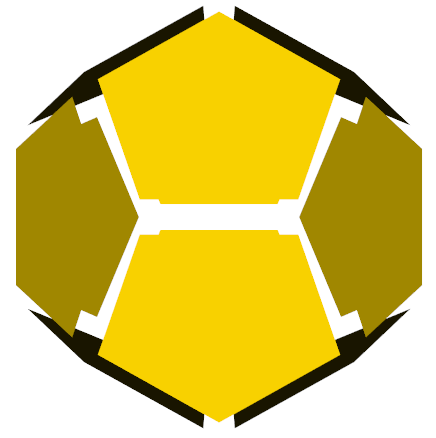warpToPoint(point, factor=0.1, absolute=True)[source]

Modify the mesh coordinates by moving the vertices towards a specified point.

Parameters
• factor (float) – value to scale displacement.

• point (list) – the position to warp towards.

• absolute (bool) – turning on causes scale factor of the new position to be one unit away from point.

Example
from vedo import *
s = Cylinder(height=3).wireframe()
pt = [4,0,0]
w = s.clone().warpToPoint(pt, factor=0.5).wireframe(False)
show(w,s, Point(pt), axes=1)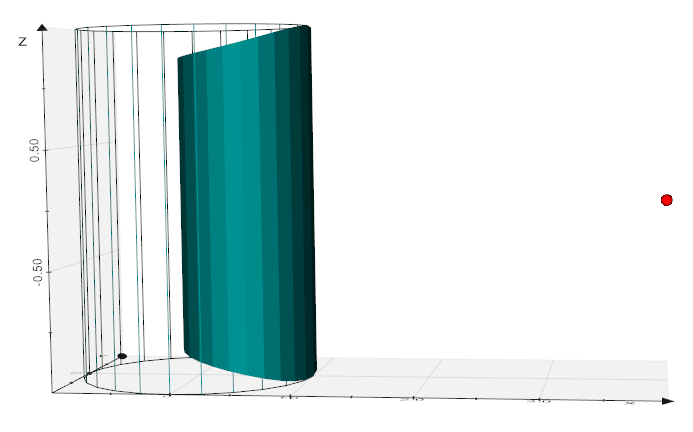wireframe(value=True)[source]

Set mesh’s representation as wireframe or solid surface. Same as mesh.wireframe().

## merge¶

vedo.mesh.merge(*meshs)[source]

Build a new mesh formed by the fusion of the polygonal meshes of the input objects. Similar to Assembly, but in this case the input objects become a single mesh.